## A uniform magnetic field passes through a horizontal circular wire loop at an angle 15.1∘ from the vertical. The magnitude of the magnetic f

Question

A uniform magnetic field passes through a horizontal circular wire loop at an angle 15.1∘ from the vertical. The magnitude of the magnetic field B changes in time according to the equation B(t)=(3.75 T)+(2.75 Ts)t+(−7.05 Ts2)t2 If the radius of the wire loop is 0.210 m, find the magnitude  of the induced emf in the loop when t=5.63 s.

in progress 0
3 months 2021-08-02T19:20:14+00:00 1 Answers 3 views 0

1. Explanation:

Given that,

A uniform magnetic field passes through a horizontal circular wire loop at an angle 15.1∘ from the vertical,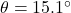The magnitude of the magnetic field B changes in time according to the equation :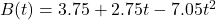Radius of the loop, r = 0.21 m

We need to find the magnitude of the induced emf in the loop when t=5.63 s. The induced emf is given by :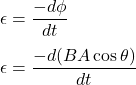B is magnetic field

A is area of cross section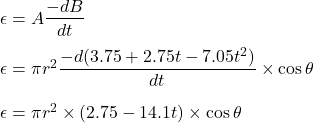At t = 5.63 seconds,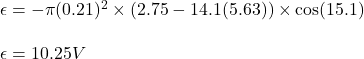So, the magnitude of induced emf in the loop when t=5.63 s is 10.25 V.